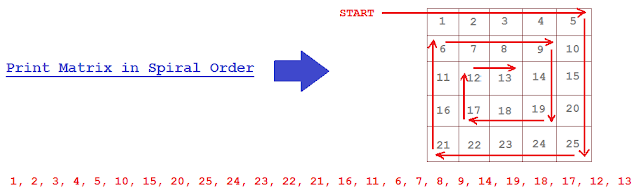# Print Matrix in Spiral form

Print Matrix in Spiral order OR
Given m*n matrix(m rows, n columns), print all elements of the matrix in spiral order OR

Print two-dimensional array in spiral order OR
How to print elements of Matrix in Spiral Format?

Given a Matrix(2D array), print it in spiral form. Lets understand the problem statement graphically and it will be more clear,### Algorithm

There are 2 ways to print matrix in Spiral order.
1. Iterative.
2. Recursive.

### In this approach, we will focus on Iterative approach.

In Iterative approach, we need to maintain 4 variables rowStart, rowLength, colStart, colLength which help in printing matrix in Spiral way.
1. Left to Right.
Move variable i from rowStart till colLength. (
Print data from first row till last column.)

2. Top to Bottom.
Move variable i from (rowStart+1) till rowLength. (Print data in last column till)
We need to start from
(rowStart+1) because, we already printed corner element in Left to Right printing and no need to include it again. Same treatment for corner elements in other directions.

3. Right to Left.
Move variable i from colLength-1 till colStart. (Print data in last row)

4. Bottom to Up.
Move variable i from rowLength-1 till rowStart. (Print data in first column)

5. After printing all 4 directions, In next iteration,
we need to start from second row and second column, so increment
(rowStart ++) and (colStart++).

we need to print till second Last column and till second Last row, so decrement
(colLength --) and

### Java Program to Print Matrix in Spiral order

```package matrix;

public class PrintMatrixInSpiralForm {

public static void main(String[] args) {
new PrintMatrixInSpiralForm();
}

public PrintMatrixInSpiralForm() {
int[][] matrix = {
{1,  2,  3,  4,  5},
{6,  7,  8,  9,  10},
{11, 12, 13, 14, 15},
{16, 17, 18, 19, 20},
{21, 22, 23, 24, 25}
};

printMatrixInSpiralWay(matrix);
}

private void printMatrixInSpiralWay(int[][] matrix){

int rowStart=0;
int rowLength=matrix.length-1;

int colStart=0;
int colLength = matrix.length-1;

while(rowStart <= rowLength && colStart <= colLength){

for (int i = rowStart; i <= colLength; i++) {
System.out.print(matrix[rowStart][i] + " ");
}

for (int j = rowStart+1; j <= rowLength; j++) {
System.out.print(matrix[j][colLength] + " ");
}

if(rowStart+1 <= rowLength){
for (int k = colLength-1; k >= colStart; k--) {
System.out.print(matrix[rowLength][k] + " ");
}
}
if(colStart+1 <= colLength){
for (int k = rowLength-1; k > rowStart; k--) {
System.out.print(matrix[k][colStart] + " ");
}
}

rowStart++;
rowLength--;
colStart++;
colLength--;
}
}
}

```
we need to check condition "if (rowStart+1 <= rowLength)" while printing from Right to Left because, if Matrix contains only 1 row then this condition will help in eliminating further iteration.

Similarly, we check "if(colStart+1 <= colLength)" while printing from Bottom to Top because, if Matrix contains only 1 Column then this condition will help in eliminating further iteration.

### You may also like to see

#### How is ambiguous overloaded method call resolved in java

Enjoy !!!!

If you find any issue in post or face any error while implementing, Please comment.